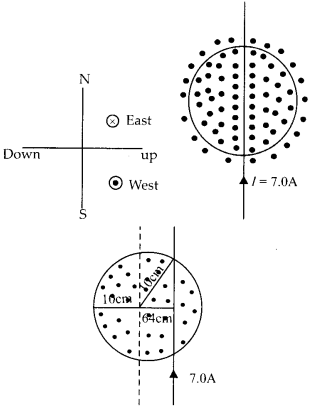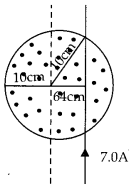Enlightened

# Question 23: NCERT Solutions for 12th Class Physics: Chapter 4-Moving Charges and Magnetism

• 0

Question 23: NCERT Solutions for 12th Class Physics: Chapter 4-Moving Charges and Magnetism

A uniform magnetic field of 1.5 T exists in a cylindrical region of radius 10.0 cm, its direction parallel to the axis along east to west. A wire carrying current of 7.0 A in the north to south direction passes through this region. What is the magnitude and direction of the force on the wire if,
(a) the wire intersects the axis,
(b) the wire is turned from N-S to northeast- northwest direction.
(c) the wire in the N-S direction is lowered from the axis by a distance of 6.0 cm?

Share

1. Solution:The magnetic field is in the direction east to west and in the cylindrical region of radius 10 cm.
(a) A current carrying wire, intersects the axis. Force on wire, F = |B| = IB (2r) = 7 × 1.5 × 2 × 1 × 10-2 F = 2.1 N Downwards
(b) By turning the wire by an angle 45° in NE and NW direction the force remain the same,/= 2.1 N Downwards
(c) Now the wire is lowered from the axis by a distance of 6.0 cm.Length of wire inside cylindrical region is 16 cm now. So, the force is F = |B| = 7 × 1.5 × 16 × 10-2 = 1.68 N, vertically downwards

Check the complete chapter with solutions.

NCERT Solutions for 12th Class Physics: Chapter 4-Moving Charges and Magnetism

• 0